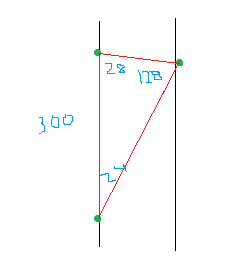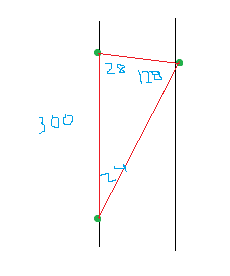# Trig Problem - Finding the width of a river

• Nobuko42

#### Nobuko42

1. An observer is next to a river and wants to calculate the distance across the river. He measures the angle between his observations of two points on the shore, one on his side and one on the other side, the angle coming out to be 28º. The distance between him and the point that is on the side side of him is 300 meters. The angle formed by him, the point on his side of the river, and the point directly on the opposite side of the river is 128º. What is the distance across the river?:

## Homework Equations

From what I can see, this is a trig type problem. I used the rule of triangles in where sin(A)/a = sin(B)/b = sin(C)/c to find the distance from point on the observer's side and the point on the opposite side of the river. I make A 28°, the a is my unknown distance, I make B 24° (180 - [128 + 28] = 24), and make b 300 meters, the distance from point and the observer on the same side. I get 346.27 meters for the distance between the two points on the shore of the rivers.

Given that the angle made by the observer, the point on the observer's side, and the point across, it appears that the two points are not directly across from one another, rather that the point across is further back then the point on the same side. What throws me off is the drawing for this problem, it shows that the observer and the point on the same side are right next to the river, and that the point across the right is directly across from each other, thus it looks like it should be making a 90° angle when taking those three points.

I make the conclusion that the observer and the point on his side are both next to each other, thus making a parallel line to the river when taking the 300 meter distance, meaning that the point across the river is further back.

With the 346.27 meter distance measured, I make a right triangle with the 346.27 being the hyposthus of the triangle. The angle of the point on the side of the observer, at the point is 52° (180 - 128 = 52), thus I use sin(52) = y/346.27 to find the distance across the river, getting y = 272.86 meter.

Here is my problem, there are multiple choice answers and none of them match my final answer. The possible answers are:

a) 89.7 m b) 346 m c) 179 m d) 6.4 x 10^-4 m

So I am unsure as to where my mistake may be, am I not reading the problem correctly as far as the placement of the three points, or making a calculation error. Assistance on this would be greatly appreciated, thank you.

1. An observer is next to a river and wants to calculate the distance across the river. He measures the angle between his observations of two points on the shore, one on his side and one on the other side, the angle coming out to be 28º. The distance between him and the point that is on the side side of him is 300 meters. The angle formed by him, the point on his side of the river, and the point directly on the opposite side of the river is 128º. What is the distance across the river?:

## Homework Equations

From what I can see, this is a trig type problem. I used the rule of triangles in where sin(A)/a = sin(B)/b = sin(C)/c to find the distance from point on the observer's side and the point on the opposite side of the river. I make A 28°, the a is my unknown distance, I make B 24° (180 - [128 + 28] = 24), and make b 300 meters, the distance from point and the observer on the same side. I get 346.27 meters for the distance between the two points on the shore of the rivers.

Given that the angle made by the observer, the point on the observer's side, and the point across, it appears that the two points are not directly across from one another, rather that the point across is further back then the point on the same side. What throws me off is the drawing for this problem, it shows that the observer and the point on the same side are right next to the river, and that the point across the right is directly across from each other, thus it looks like it should be making a 90° angle when taking those three points.

I make the conclusion that the observer and the point on his side are both next to each other, thus making a parallel line to the river when taking the 300 meter distance, meaning that the point across the river is further back.

With the 346.27 meter distance measured, I make a right triangle with the 346.27 being the hyposthus of the triangle. The angle of the point on the side of the observer, at the point is 52° (180 - 128 = 52), thus I use sin(52) = y/346.27 to find the distance across the river, getting y = 272.86 meter.

Here is my problem, there are multiple choice answers and none of them match my final answer. The possible answers are:

a) 89.7 m b) 346 m c) 179 m d) 6.4 x 10^-4 m

So I am unsure as to where my mistake may be, am I not reading the problem correctly as far as the placement of the three points, or making a calculation error. Assistance on this would be greatly appreciated, thank you.
If there's an illustration, can you post it? That would be helpful.Now that you have the picture, you can do:
$\frac{300}{sin128}=\frac{x}{sin28}$

That will give you the answer, but this is kind of broad because you can also find the distance directly across from the person, not just the hypotenuse, so it might be that answer too..Now that you have the picture, you can do:
$\frac{300}{sin128}=\frac{x}{sin28}$

That will give you the answer, but this is kind of broad because you can also find the distance directly across from the person, not just the hypotenuse, so it might be that answer too..

The drawing iraid made is kind of like what the problem says, except that the observer is the one on the bottom, and the two points appear to be directly across. However the drawing isn't to scale in anyway, as the angle between the observer, the point on his side, and the point across looks like 90 degrees, so it doesn't really help in regards to where each one is. I am thinking it might be the observer isn't on the shore, but somewhere down some, due to the problem saying directly across, but the drawing shows otherwise :-( on my cell so can't draw till I get home

Sorry it's not to scale, but I think that's right. The observer is the bottom dot. The answer I get is ~179 m..

Oh I didn't mean your drawing, I meant the one in the problem I have. I think your angles may not be placed correctly, as the problem says "He measures the angle between his observations of two points on the shore, one on his side and one on the other side, the angle coming out to be 28º" I think that means that the 28° is at the point of the observer, not on the point of the same side as the observer. The wording is so vague on some parts which is what is throwing me off, one part saying the two points are directly across from each other, while the other says that the observer is next to the river :\